Courses

# NCERT Solutions(Part- 3)- Practical Geometry Class 8 Notes | EduRev

## Class 8 : NCERT Solutions(Part- 3)- Practical Geometry Class 8 Notes | EduRev

The document NCERT Solutions(Part- 3)- Practical Geometry Class 8 Notes | EduRev is a part of the Class 8 Course Class 8 Mathematics by VP Classes.
All you need of Class 8 at this link: Class 8

Question 1. How will you construct a rectangle PQRS if you know only the lengths PQ and QR?

Solution: A rectangle can be constructed by taking PQ as the length.

Making an ∠90° at Q and cutting off QR, the breadth the ray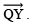Remaining two points R and S can be located by taking P and R as centres and radii as QR and PQ respectively draw arcs to intersect at S. Thus, PQRS is the required parallelogram.Question 2. Construct the kite EASY if AY = 8 cm, EY = 4 cm and SY = 6 cm. Which properties of the kite did you use in the process?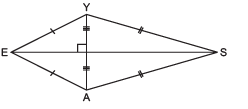Solution: Following properties have been used in constructing the KITE:

(i) Diagonals are at right angles.

(ii) One of the diagonal bisects the other.

(iii) Pairs of consecutive sides are equal.

Steps of construction:

I. Draw a line segment AY = 8 cm.
II. Draw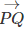, the perpendicular bisector of AY such that it meets AY at O.
III. We cannot locate a point E on PQ at 4 cm from Y and A, i.e. EY = 4 cm = EA is not possible. It is possible only when E and O coincide. In that case the kite does not exist.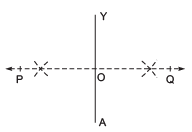EXERCISE 4.5
Question: Draw the following.
1. The square READ with RE = 5.1 cm.
2. A rhombus whose diagonals are 5.2 cm and 6.4 cm long.
3. A rectangle with adjacent sides of lengths 5 cm and 4 cm.
4. A parallelogram OKAY where OK = 5.5 cm and KA = 4.2 cm.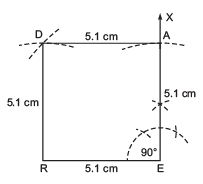Solution:1. Steps of construction:

I. Draw a line segment RE = 5.1 cm.

II. At E, draw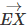, such that ∠REX = 90°.

III. From, cut-off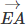= 5.1 cm.

IV. With centre at A, draw an arc above RE of radius = 5.1 cm.

V. With centre at R, and radius = 5.1 cm, draw another arc to intersect the previous arc at D.

VI. Join DA and DR.

Thus, READ is the required square.

2. Steps of construction: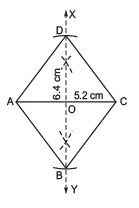Note: The diagonals of a rhombus bisect each other at right angles.

I. Draw a line segment AC = 5.2 cm.

II. Draw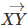, the perpendicular bisector of AC.

III. From XY, cut-off OD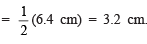IV. Similarly, cut-off OB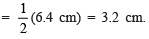V. Join AD, DC, CB and BA.

Thus, ABCD is the required rhombus.

3. Steps of construction: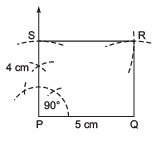I. Draw a line segment PQ = 5 cm.

II. At P, draw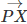, such that ∠QPX = 90°

III. From, cut-off PS = 4 cm.

IV. With centre at 5 and radius = 5 cm, mark an arc towards Q.

V. With centre Q and radius = 4 cm, mark an arc to intersect the previous arc at R.

VI. Join RQ and RS

Thus, PQRS is the required rectangle.

4. Steps of construction: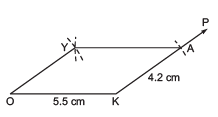I. Draw a line segment OK = 5.5 cm.

II. At K, draw a ray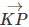.

III. From, cut-off KA = 4.2 cm.

IV. With centre at A and radius = 5.5 cm, draw an arc above OK.

V. With centre O and radius = 4.2 cm, draw another arc to intersect the previous arc at Y.

VI. Join YO and YA.

Thus, OKAY is the required parallelogram.

Offer running on EduRev: Apply code STAYHOME200 to get INR 200 off on our premium plan EduRev Infinity!

89 docs|16 tests

,

,

,

,

,

,

,

,

,

,

,

,

,

,

,

,

,

,

,

,

,

;